You are currently offline. Some features of the site may not work correctly.

# Binomial type

Known as: Binomial
In mathematics, a polynomial sequence, i.e., a sequence of polynomials indexed by { 0, 1, 2, 3, ... } in which the index of each polynomial equals… Expand
Wikipedia

## Papers overview

Semantic Scholar uses AI to extract papers important to this topic.
2014
2014
We present various constructions of sequences of polynomials satisfying the Binomial Theorem in finite characteristic based on… Expand
Is this relevant?
2008
2008
This paper concerns the study of the Bell polynomials and the binomial type sequences. We mainly establish some relations tied to… Expand
Is this relevant?
2007
2007
• Empirical Software Engineering
• 2007
• Corpus ID: 8790677
A new approach to software reliability modeling is discussed where variables indirectly related with software reliability are… Expand
•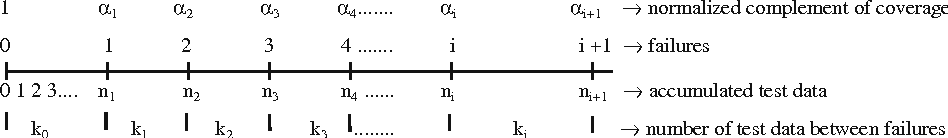•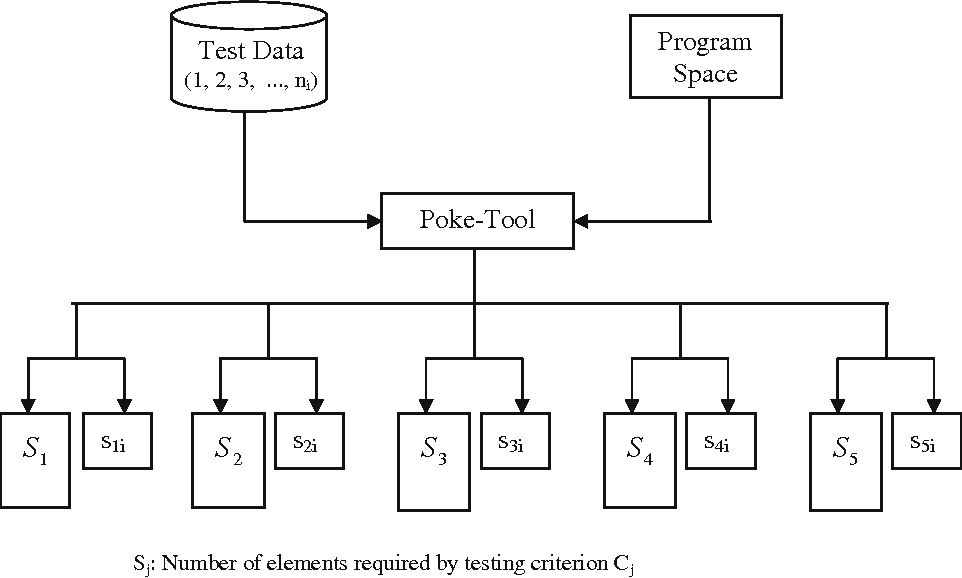•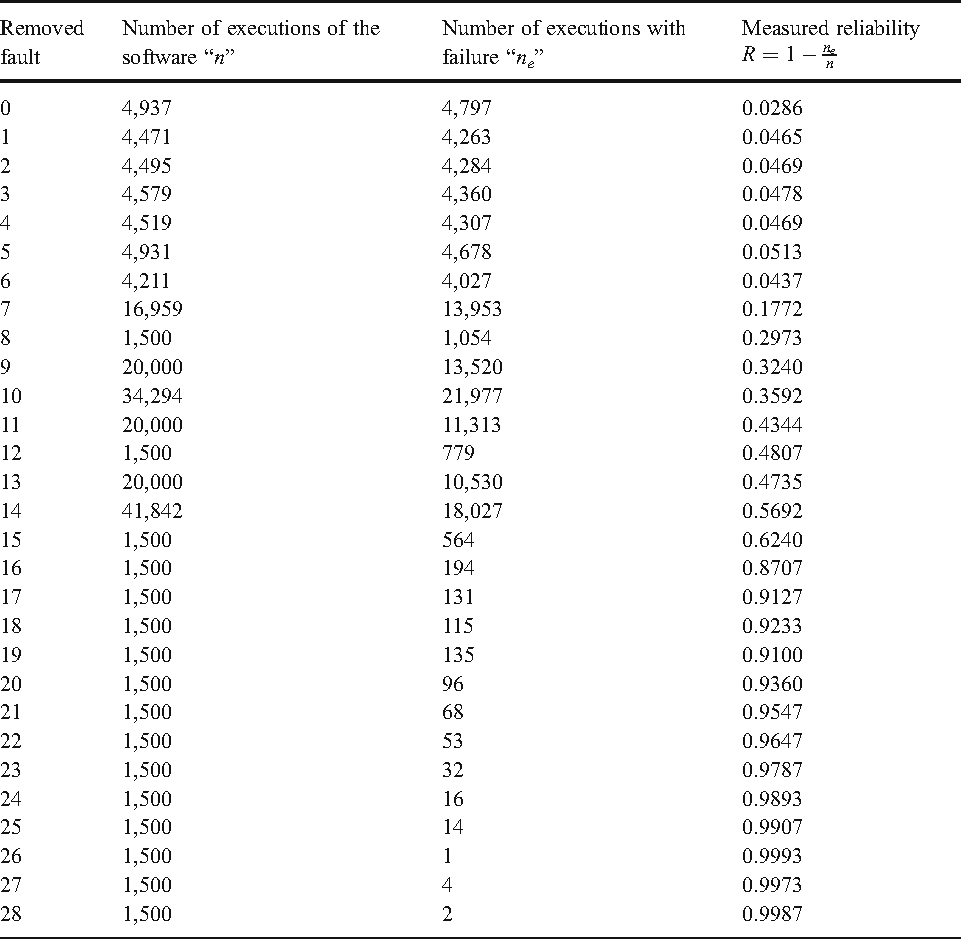•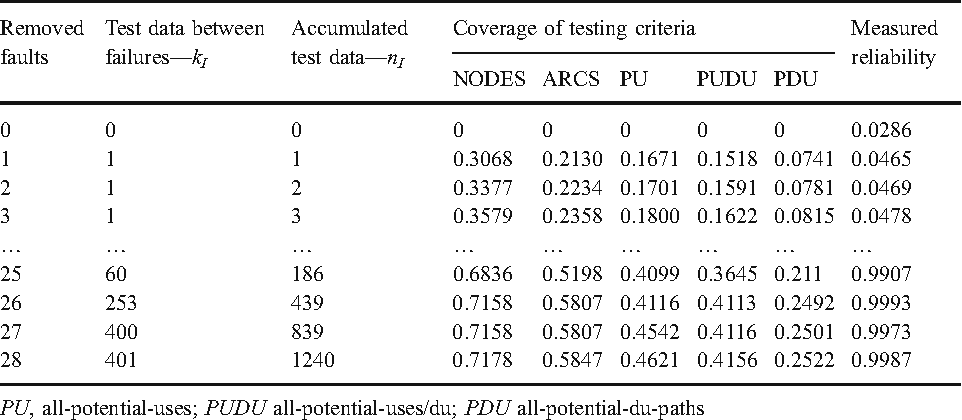•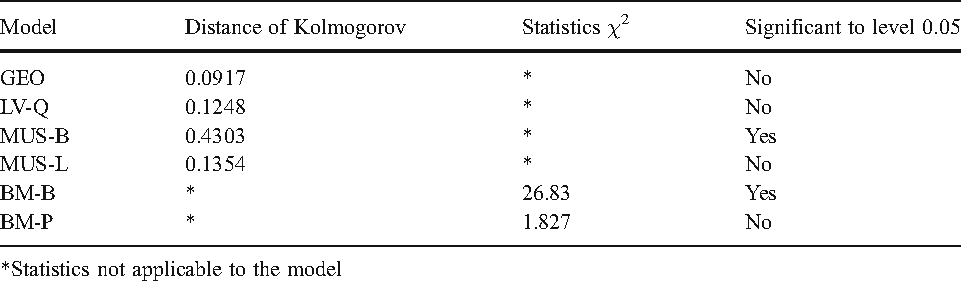Is this relevant?
2004
2004
• 2004
• Corpus ID: 41946254
We derive and characterize a new mixed-risk distribution of the total number of Bernoulli outcomes (e.g, failures) for non… Expand
•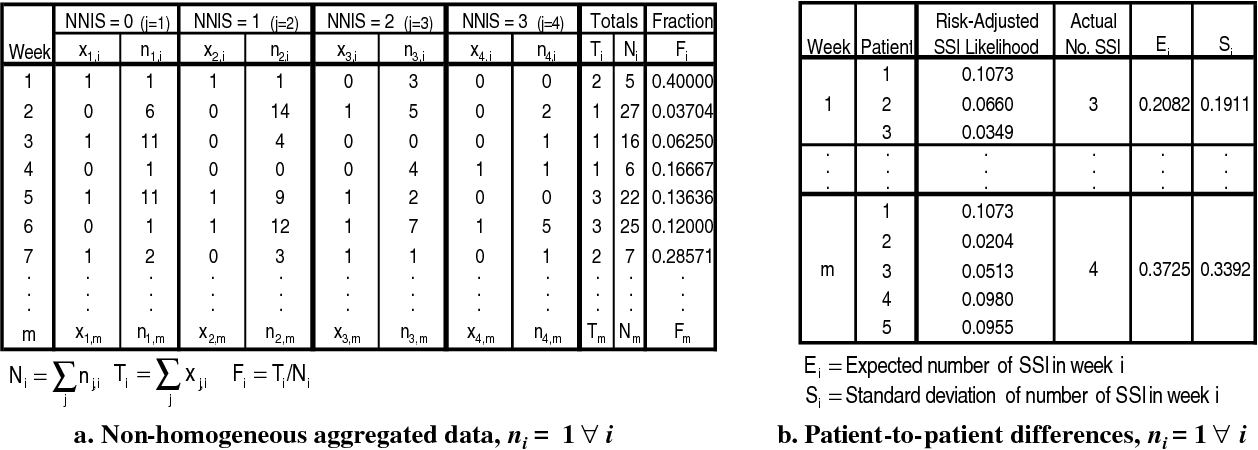•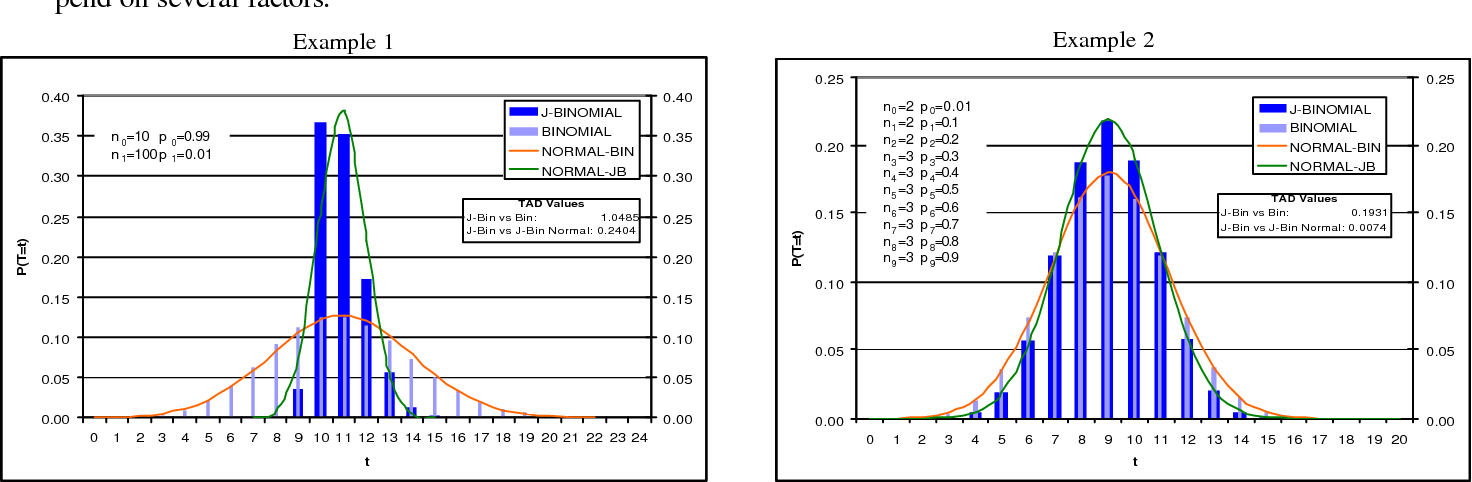•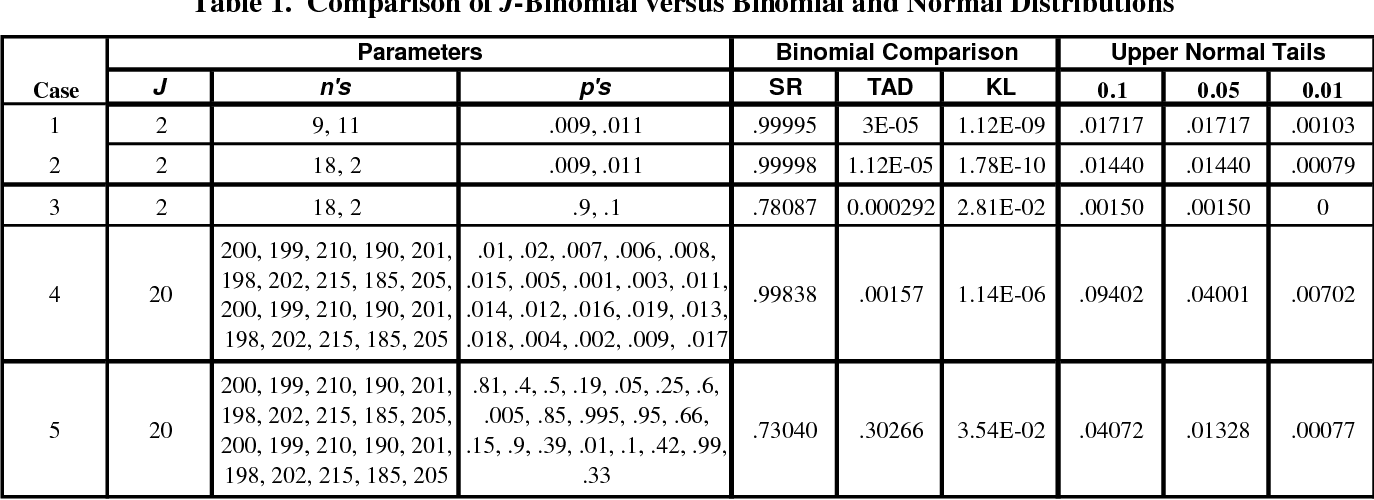•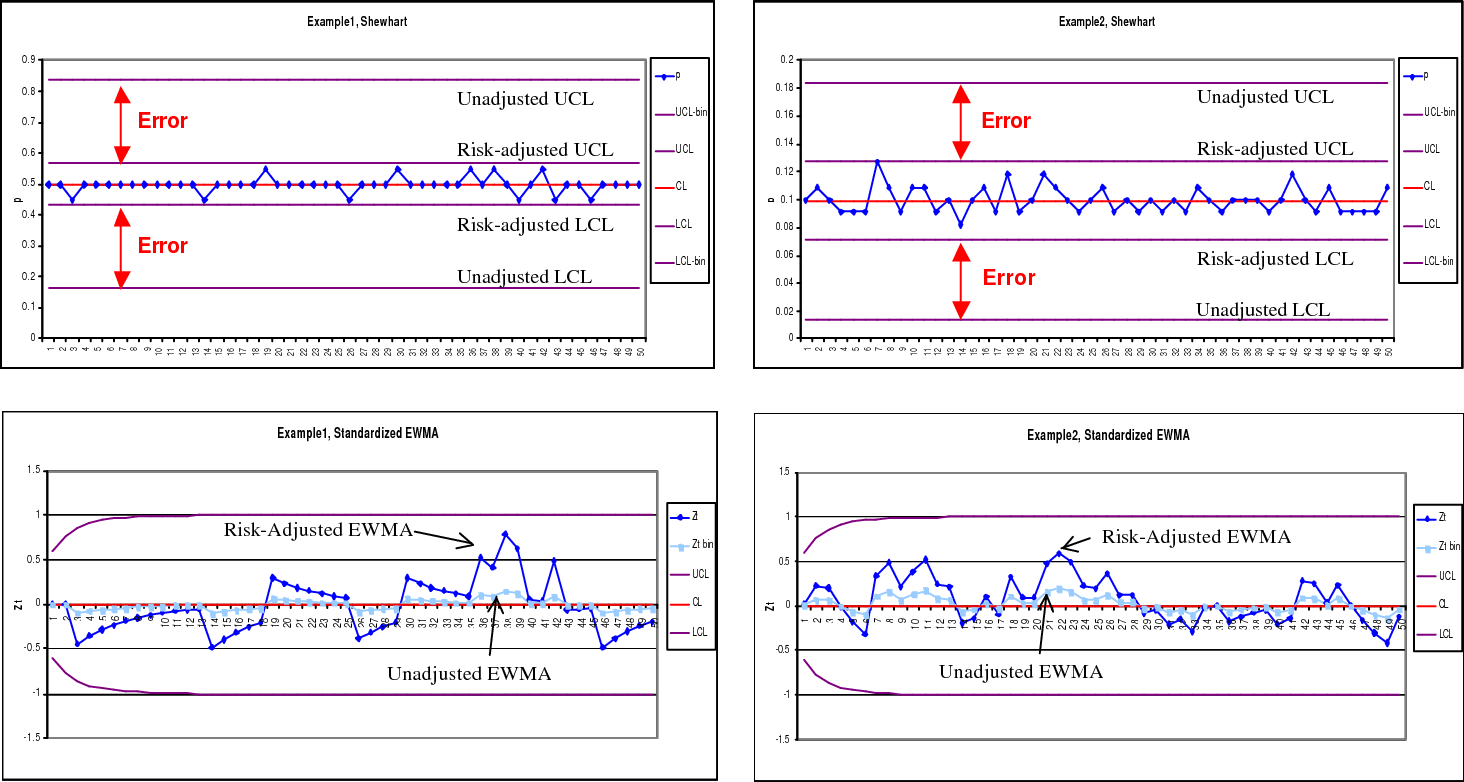•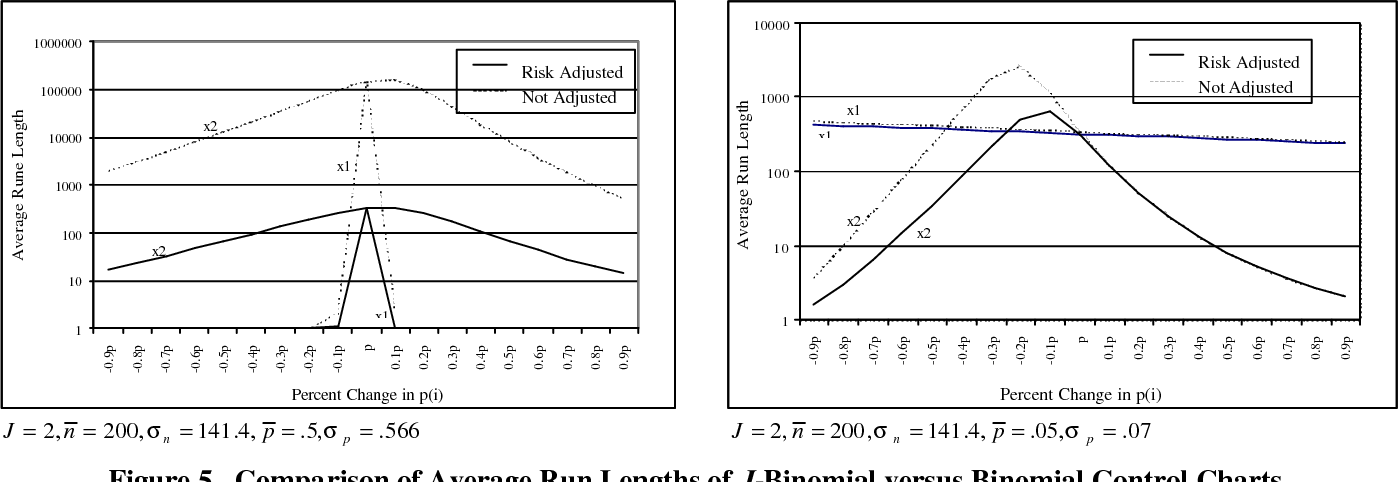Is this relevant?
1997
1997
• 1997
• Corpus ID: 55738351
L’accès aux archives de la revue « Annali della Scuola Normale Superiore di Pisa, Classe di Scienze » (http://www.sns.it/it… Expand
Is this relevant?
1997
1997
We generalize the binomial formula for Jack polynomials proved in [OO2] and consider some applications. §1 Binomial formula… Expand
•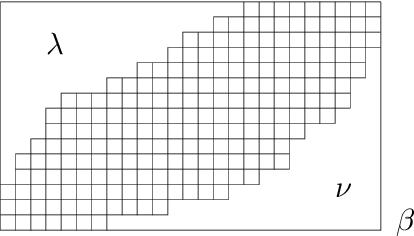•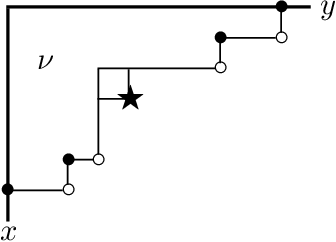Is this relevant?
1996
1996
• 1996
• Corpus ID: 121141052
Abstract We find all sequences of polynomials ( p n ) n ⩾0 with persistent roots (i.e., p n ( x )= c n ( x − r 1 )( x − r 2… Expand
Is this relevant?
1991
1991
Sequences of binomial type are thoroughly structured polynomial sequences. We view them as being parametrized by their delta… Expand
•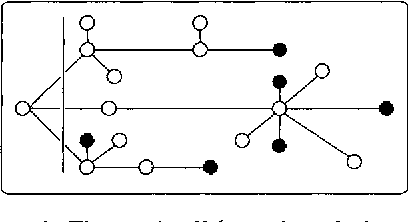Is this relevant?
1977
1977
Abstract We introduce the problem of establishing a central limit theorem for the coefficients of a sequence of polynomials Pn(x… Expand
Is this relevant?
1973
1973
The theory of binomial enumeration leads to sequences of functions of binomial type which are not polynomials. The results of… Expand
Is this relevant?